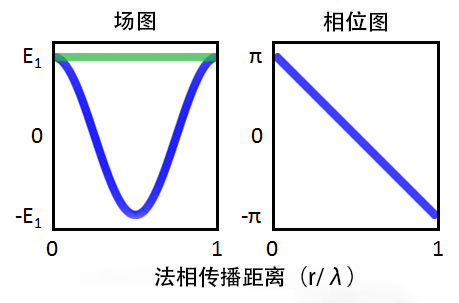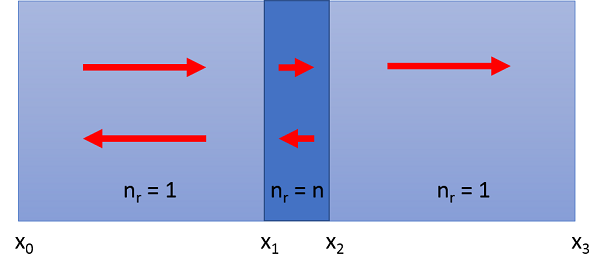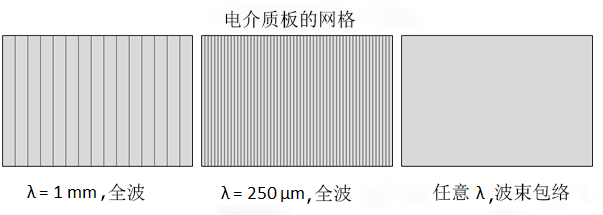# 比较两个高频建模接口

2016年 5月 26日

“工欲善其事，必先利其器”。在进行高频电磁仿真时，选择适合的接口是十分重要的。在这篇博客文章中，我们以空气中平面波入射到介电板为例，使用了两种不同的方法对其进行求解，并根据仿真结果阐释电磁波，频域 接口与电磁波，波束包络 接口在实际应用中的差异和各自的优势。

### 在两个电磁接口中对自由空间剖分网格### 在 COMSOL Multiphysics 中对电介质板剖分网格\phi\left(\mathbf{r}\right) = k_0\cdot\left(x-x_0\right)
\phi\left(\mathbf{r}\right) = k_0\cdot\left(\left(x_1-x_0\right) + n\cdot\left(x-x_1\right)\right)
\phi\left(\mathbf{r}\right) = k_0\cdot\left(\left(x_1-x_0\right) + n\cdot\left(x_2-x_1\right) + \left(x-x_2\right)\right)1 mm 4,134 74
250 µm 16,444 74

### 选择最恰当的波矢

\mathbf{E}\left(\mathbf{r}\right)e^{-j\mathbf{k_G}\cdot\mathbf{r}} = \mathbf{E_1}e^{-j\mathbf{k}\cdot\mathbf{r}} + \mathbf{E_2}e^{j\mathbf{k}\cdot\mathbf{r}}

\mathbf{E}\left(\mathbf{r}\right) = \mathbf{E_1}e^{-j\left(\mathbf{k-k_G}\right)\cdot\mathbf{r}} + \mathbf{E_2}e^{j\left(\mathbf{k+k_G}\right) \cdot\mathbf{r}}

\mathbf{E}\left(\mathbf{r}\right) = \mathbf{E_1} + \mathbf{E_2}e^{j\left(\mathbf{k+k_G}\right)\cdot\mathbf{r}}.

\lambda_{eff} = \frac{2\pi}{\left|\mathbf{k+k_G}\right|} = \frac{2\pi}{2\left|\mathbf{k}\right|} = \frac{\lambda}{2}

### 更多资源

• 浏览“COMSOL 博客”，阅读更多关于 电气建模的文章
• 观看以下视频：
• 参加当地的培训活动，提升针对电磁学的建模能力
• 欢迎联系我们，咨询您在建模中遇到的问题